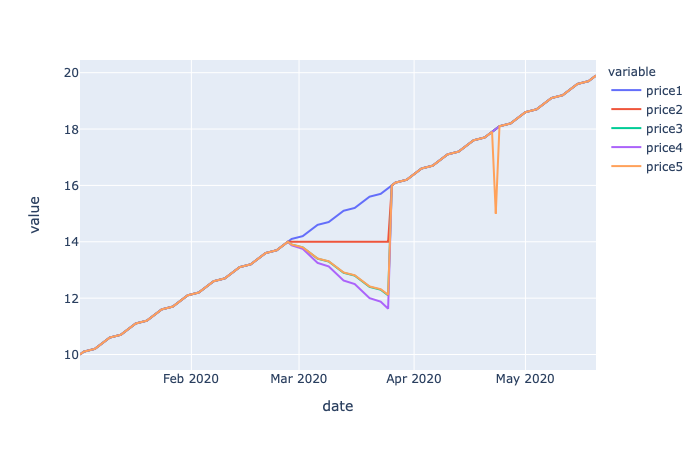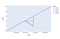# Sharpe Ratio, Sorino Ratio and Calmar RatioWe are going to examine the deficiencies of Sharpe ratio, and how we can complement it with Sorino Ratio and Calmar Ratio to gain a clearer picture of the performance of a portfolio.

In this short story, we are going to examine the deficiencies of Sharpe ratio, and how we can complement it with Sorino Ratio and Calmar Ratio to gain a clearer picture of the performance of a portfolio.

In portfolio performance analysis, sharpe ratio is the usually the first number that people look at. However, it does not tell us the whole story (nothing does…). So, let’s spend some time looking at a few more metrics that can be very helpful at times.

### Sharpe Ratio Revisited

Sharpe ratio is the ratio of average return divided by the standard deviation of returns annualized. We had an introduction to it in a previous story.

Let’s take a look at it again with a test price time series.

``````import pandas as pd
import numpy as np

from pandas.tseries.offsets import BDay

def daily_returns(prices):

res = (prices/prices.shift(1) - 1.0)[1:]
res.columns = ['return']

return res

def sharpe(returns, risk_free=0):

def test_price1():

start_date = pd.Timestamp(2020, 1, 1) + BDay()

len = 100

bdates = [start_date + BDay(i) for i in range(len)]
price = [10.0 + i/10.0 for i in range(len)]

return pd.DataFrame(data={'date': bdates,
'price1': price}).set_index('date')

def test_price2():

start_date = pd.Timestamp(2020, 1, 1) + BDay()

len = 100

bdates = [start_date + BDay(i) for i in range(len)]
price = [10.0 + i/10.0 for i in range(len)]

price[40:60] = [price for i in range(20)]

return pd.DataFrame(data={'date': bdates,
'price2': price}).set_index('date')

def test_price3():

start_date = pd.Timestamp(2020, 1, 1) + BDay()

len = 100

bdates = [start_date + BDay(i) for i in range(len)]
price = [10.0 + i/10.0 for i in range(len)]

price[40:60] = [price - i/10.0 for i in range(20)]

return pd.DataFrame(data={'date': bdates,
'price3': price}).set_index('date')

def test_price4():

start_date = pd.Timestamp(2020, 1, 1) + BDay()

len = 100

bdates = [start_date + BDay(i) for i in range(len)]
price = [10.0 + i/10.0 for i in range(len)]

price[40:60] = [price - i/8.0 for i in range(20)]

return pd.DataFrame(data={'date': bdates,
'price4': price}).set_index('date')

price1 = test_price1()
return1 = daily_returns(price1)

price2 = test_price2()
return2 = daily_returns(price2)

price3 = test_price3()
return3 = daily_returns(price3)

price4 = test_price4()
return4 = daily_returns(price4)

print('price1')
print(f'sharpe: {sharpe(return1)}')

print('price2')
print(f'sharpe: {sharpe(return2)}')

print('price3')
print(f'sharpe: {sharpe(return3)}')

print('price4')
print(f'sharpe: {sharpe(return4)}')
``````## Data Visualization With Python | Data Visualization | Python For Data Science

🔥To access the slide deck used in this session for Free, click here: https://bit.ly/GetPDF_DataV_P 🔥 Great Learning brings you this live session on 'Data Vis...

## Applied Data Science with Python Certification Training Course -IgmGuru

Master Applied Data Science with Python and get noticed by the top Hiring Companies with IgmGuru's Data Science with Python Certification Program. Enroll Now

## An introduction to exploratory data analysis in python

Many a time, I have seen beginners in data science skip exploratory data analysis (EDA) and jump straight into building a hypothesis function or model. In my opinion, this should not be the case.

## Exploratory Data Analysis is a significant part of Data Science

Data science is omnipresent to advanced statistical and machine learning methods. For whatever length of time that there is data to analyse, the need to investigate is obvious.

## Python For Data Science | Python For Data Analysis

Python for Data Science, you will be working on an end-to-end case study to understand different stages in the data science life cycle. This will mostly deal with "data manipulation" with pandas and "data visualization" with seaborn. After this, an ML model will be built on the dataset to get predictions. You will learn about the basics of the sci-kit-learn library to implement the machine learning algorithm.